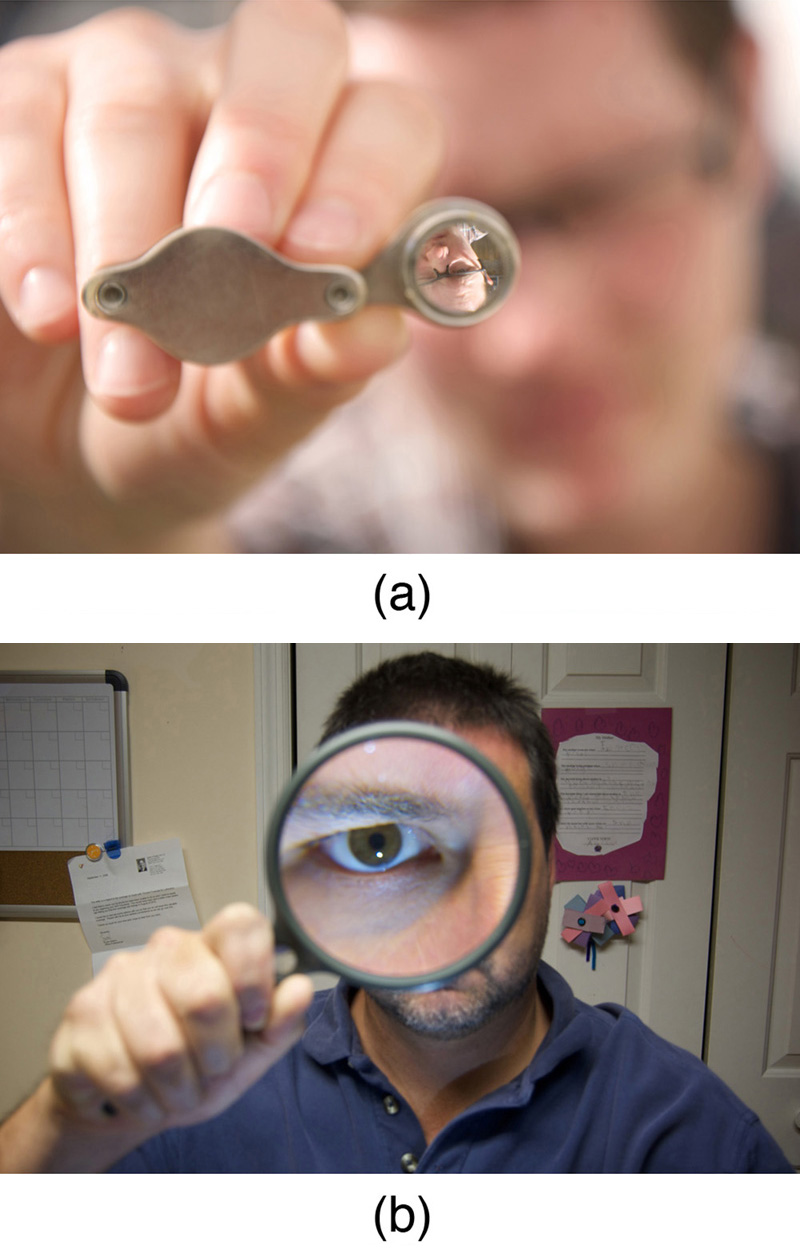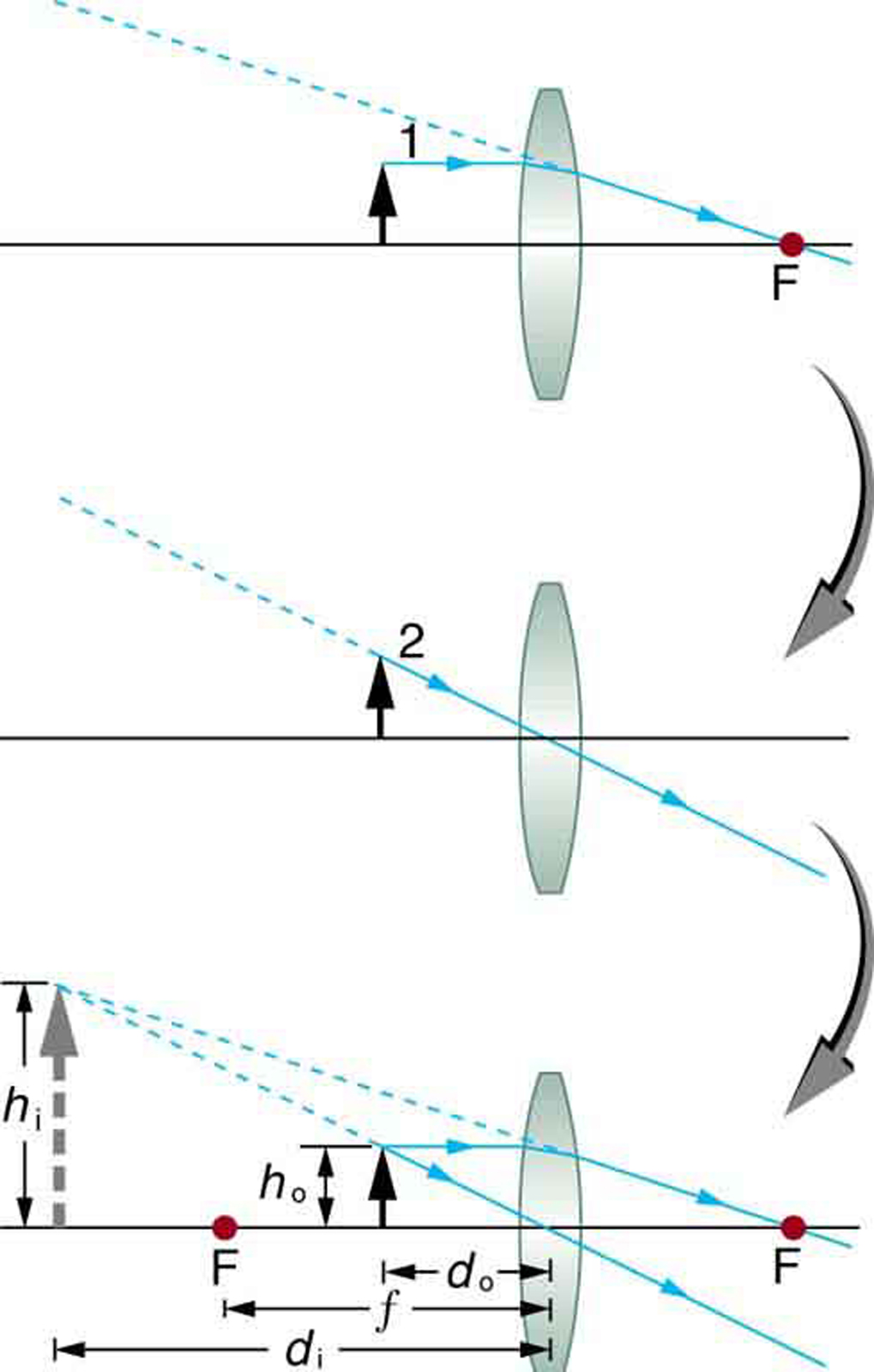# 25.6 Image formation by lenses  (Page 7/19)

 Page 7 / 19(a) When a converging lens is held farther away from the face than the lens’s focal length, an inverted image is formed. This is a case 1 image. Note that the image is in focus but the face is not, because the image is much closer to the camera taking this photograph than the face. (credit: DaMongMan, Flickr) (b) A magnified image of a face is produced by placing it closer to the converging lens than its focal length. This is a case 2 image. (credit: Casey Fleser, Flickr)

[link] uses ray tracing to show how an image is formed when an object is held closer to a converging lens than its focal length. Rays coming from a common point on the object continue to diverge after passing through the lens, but all appear to originate from a point at the location of the image. The image is on the same side of the lens as the object and is farther away from the lens than the object. This image, like all case 2 images, cannot be projected and, hence, is called a virtual image    . Light rays only appear to originate at a virtual image; they do not actually pass through that location in space. A screen placed at the location of a virtual image will receive only diffuse light from the object, not focused rays from the lens. Additionally, a screen placed on the opposite side of the lens will receive rays that are still diverging, and so no image will be projected on it. We can see the magnified image with our eyes, because the lens of the eye converges the rays into a real image projected on our retina. Finally, we note that a virtual image is upright and larger than the object, meaning that the magnification is positive and greater than 1.Ray tracing predicts the image location and size for an object held closer to a converging lens than its focal length. Ray 1 enters parallel to the axis and exits through the focal point on the opposite side, while ray 2 passes through the center of the lens without changing path. The two rays continue to diverge on the other side of the lens, but both appear to come from a common point, locating the upright, magnified, virtual image. This is a case 2 image.

## Virtual image

An image that is on the same side of the lens as the object and cannot be projected on a screen is called a virtual image.

## Image produced by a magnifying glass

Suppose the book page in [link] (a) is held 7.50 cm from a convex lens of focal length 10.0 cm, such as a typical magnifying glass might have. What magnification is produced?

Strategy and Concept

We are given that ${d}_{\text{o}}=7\text{.}\text{50 cm}$ and $f=\text{10}\text{.}\text{0 cm}$ , so we have a situation where the object is placed closer to the lens than its focal length. We therefore expect to get a case 2 virtual image with a positive magnification that is greater than 1. Ray tracing produces an image like that shown in [link] , but we will use the thin lens equations to get numerical solutions in this example.

Solution

To find the magnification $m$ , we try to use magnification equation, $m={\mathrm{–d}}_{\text{i}}/{d}_{\text{o}}$ . We do not have a value for ${d}_{\text{i}}$ , so that we must first find the location of the image using lens equation. (The procedure is the same as followed in the preceding example, where ${d}_{\text{o}}$ and $f$ were known.) Rearranging the magnification equation to isolate ${d}_{\text{i}}$ gives

#### Questions & Answers

A spherica, concave shaving mirror has a radius of curvature of 32 cm .what is the magnification of a persons face. when it is 12cm to the left of the vertex of the mirror
A weather vane is some sort of directional arrow parallel to the ground that may rotate freely in a horizontal plane. A typical weather vane has a large cross-sectional area perpendicular to the direction the arrow is pointing, like a “One Way” street sign. The purpose of the weather vane is to indicate the direction of the wind. As wind blows pa
If a prism is fully imersed in water then the ray of light will normally dispersed or their is any difference?
the same behavior thru the prism out or in water bud abbot
Ju
If this will experimented with a hollow(vaccum) prism in water then what will be result ?
Anurag
What was the previous far point of a patient who had laser correction that reduced the power of her eye by 7.00 D, producing a normal distant vision power of 50.0 D for her?
What is the far point of a person whose eyes have a relaxed power of 50.5 D?
Jaydie
What is the far point of a person whose eyes have a relaxed power of 50.5 D?
Jaydie
A young woman with normal distant vision has a 10.0% ability to accommodate (that is, increase) the power of her eyes. What is the closest object she can see clearly?
Jaydie
29/20 ? maybes
Ju
In what ways does physics affect the society both positively or negatively
how can I read physics...am finding it difficult to understand...pls help
try to read several books on phy don't just rely one. some authors explain better than other.
Ju
And don't forget to check out YouTube videos on the subject. Videos offer a different visual way to learn easier.
Ju
hope that helps
Ju
I have a exam on 12 february
what is velocity
Jiti
the speed of something in a given direction.
Ju
what is a magnitude in physics
Propose a force standard different from the example of a stretched spring discussed in the text. Your standard must be capable of producing the same force repeatedly.
What is meant by dielectric charge?
what happens to the size of charge if the dielectric is changed?
omega= omega not +alpha t derivation
u have to derivate it respected to time ...and as w is the angular velocity uu will relace it with "thita × time""
Abrar
do to be peaceful with any body
the angle subtended at the center of sphere of radius r in steradian is equal to 4 pi how?
if for diatonic gas Cv =5R/2 then gamma is equal to 7/5 how?
Saeed
define variable velocityByByByByBy Nick Swain